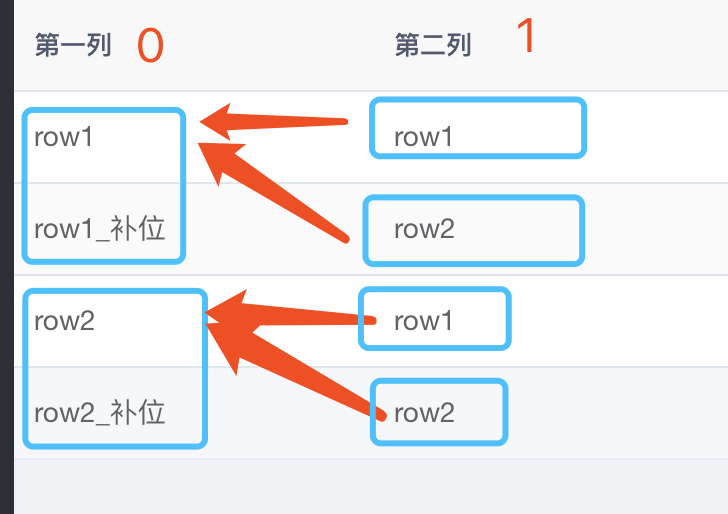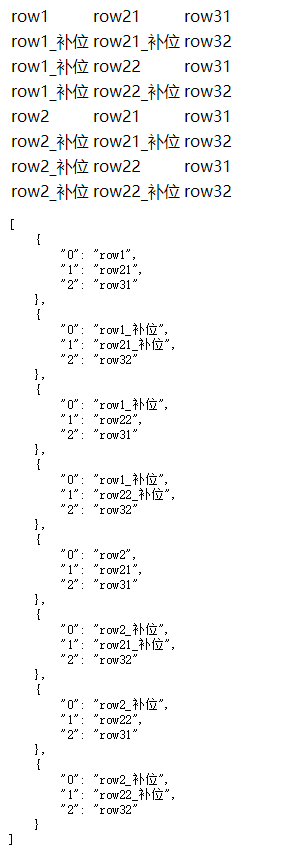2021-01-13 00:05

# vue+element类似动态横向树形表格生成问题。

50

``````<template>
<div>
<template>
<el-table
:data="tableData"
stripe
style="width: 100%">
<el-table-column
prop="0"
label="第一列"
width="180">
</el-table-column>
<el-table-column
prop="1"
label="第二列"
width="180">
</el-table-column>

</el-table>
</template>
</div>
</template>

<script>
export default {
name: "index2",
data() {
return {
"logicData": [
{
"name": "第一列",
"id": 0,
"rowList": [
{
"id": 0,
"value": "row1"
},
{
"id": 1,
"value": "row2"
}
]
},
{
"name": "第二列",
"id": 1,
"rowList": [
{
"id": 0,
"value": "row1"
},
{
"id": 1,
"value": "row2"
}
]
}
],

tableData:[
{
0:"row1",
1:"row1"
},
{
0:"row1_补位",
1:"row2"
},
{
0:"row2",
1:"row1"
},
{
0:"row2_补位",
1:"row2"
}
]
}
}
}
</script>

<style scoped>

</style>
``````logicData是一个可动态添加的数组，数组中每次加一个对象，表格就增加一列。表格的列prop绑定对象里id属性的值。如效果图中的0和1就是logicData中两个对象的id值

```rowList是当前对象对应的列的表格值。第一列的值有row1和row2.所以第一列有两行，如图。但是每行下有个补位值row1_补位和row_2补位。
```

• 点赞
• 收藏
• 复制链接分享

#### 4条回答

•点赞 评论 复制链接分享
• 前辈的解采纳了，能简单解析下思路吗，没看懂，我晕

点赞 评论 复制链接分享
• 感谢解答，晚上验证下作者的回答，可以就采纳了，酬金支付

点赞 评论 复制链接分享
• ``````<!doctype html>
<html lang="en">
<meta charset="UTF-8" />
<meta http-equiv="X-UA-Compatible" content="IE=edge,chrome=1" />
<title> 页面名称 </title>
<script src="https://cdn.jsdelivr.net/npm/vue/dist/vue.js"></script>
<body>
<div id="app">
<table>
<tr v-for="obj in tableData">
<td v-for="v in obj">{{v}}</td>
</tr>
</table>

<pre>
{{JSON.stringify(tableData,null,4)}}
</pre>
</div>

<script type="text/javascript">
new Vue({
el: '#app',
data() {
return {
"logicData": [{
"name": "第一列",
"id": 0,
"rowList": [{
"id": 0,
"value": "row1"
}, {
"id": 1,
"value": "row2"
}]
}, {
"name": "第二列",
"id": 1,
"rowList": [{
"id": 0,
"value": "row21"
}, {
"id": 1,
"value": "row22"
}]
}, {
"name": "第三列",
"id": 2,
"rowList": [{
"id": 0,
"value": "row31"
}, {
"id": 1,
"value": "row32"
}]
}]
}
},
computed: {
tableData() {
var arr = [{}];
for (var i = this.logicData.length-1; i >= 0; i--) {
var t = this.logicData[i];
ta = [];
t.rowList.forEach(g=>{
arr.forEach((p,j)=>{
var obj = Object.assign({}, p);
if (j==0)
obj[t.id] = g.value;
else
obj[t.id] = g.value + "_补位";
ta.push(obj);
});
});
arr = ta;
}
return arr;
}
}

});
</script>

</body>
</html>``````
点赞 评论 复制链接分享